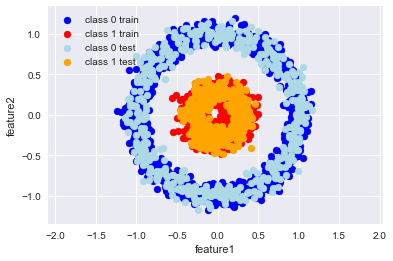In :
X,y = make_circles(n_samples = 3000, noise = 0.08, factor=0.3)
#X,y = make_moons(n_samples = 3000, noise = 0.08)
X0 = []
X1 = []
for i in range(len(y)):
if y[i] == 0:
X0.append(X[i,:])
else:
X1.append(X[i,:])

X0_np = np.array(X0)
X1_np = np.array(X1)

X0_train = X0_np[:1000,:].T # we want X to be made of examples which are stacked horizontally
X0_test = X0_np[1000:,:].T
X1_train = X1_np[:1000,:].T
X1_test = X1_np[1000:,:].T

X_train = np.hstack([X0_train,X1_train]) # all training examples
y_train=np.zeros((1,2000))
y_train[0, 1000:] = 1
X_test = np.hstack([X0_test,X1_test]) # all test examples
y_test=np.zeros((1,1000))
y_test[0, 500:] = 1

plt.scatter(X0_train[0,:],X0_train[1,:], color = 'b', label = 'class 0 train')
plt.scatter(X1_train[0,:],X1_train[1,:], color = 'r',  label = 'class 1 train')
plt.scatter(X0_test[0,:],X0_test[1,:], color = 'LightBlue', label = 'class 0 test')
plt.scatter(X1_test[0,:],X1_test[1,:], color = 'Orange', label = 'class 1 test')
plt.xlabel('feature1')
plt.ylabel('feature2')
plt.legend()
plt.axis('equal')
plt.show()

# we will plot shapes of training and test set to make sure that they were made by stacking examoles horizontally
# so in every column of these matrices there is one examples
# in two rows there are features (feature1 is ploted on the x-axis, and feature2 is ploted on the y-axis)
print('Shape of X_train set is %i x %i.'%X_train.shape)
print('Shape of X_test set is %i x %i.'%X_test.shape)
# labels for these examples are in y_train and y_test
print('Shape of y_train set is %i x %i.'%y_train.shape)
print('Shape of y_test set is %i x %i.'%y_test.shape)Shape of X_train set is 2 x 2000.
Shape of X_test set is 2 x 1000.
Shape of y_train set is 1 x 2000.
Shape of y_test set is 1 x 1000.